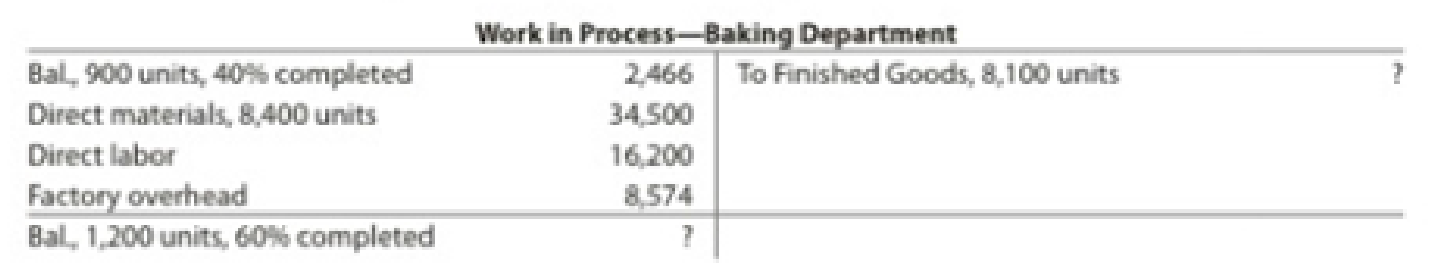# Equivalent units of production and related costs The charges to Work in Process—Baking Department for a period as well as information concerning production are as follows. The Baking Department uses the weighted average method, and all direct materials are placed in process during production. Determine the following: a. The number of whole units to be accounted for and to be assigned costs b. The number of equivalent units of production c. The cost per equivalent unit d. The cost of units transferred to Finished Goods e. The cost of units in ending Work in Process### Financial And Managerial Accounting

15th Edition
WARREN + 1 other
Publisher: Cengage Learning,
ISBN: 9781337902663### Financial And Managerial Accounting

15th Edition
WARREN + 1 other
Publisher: Cengage Learning,
ISBN: 9781337902663

#### Solutions

Chapter
Section
Chapter 17, Problem 24E
Textbook Problem

## Equivalent units of production and related costsThe charges to Work in Process—Baking Department for a period as well as information concerning production are as follows. The Baking Department uses the weighted average method, and all direct materials are placed in process during production.Determine the following: a. The number of whole units to be accounted for and to be assigned costs b. The number of equivalent units of production c. The cost per equivalent unit d. The cost of units transferred to Finished Goods e. The cost of units in ending Work in Process

Expert Solution

A.

To determine

Calculate the number of whole units to be accounted, and the units to be assigned costs.

### Explanation of Solution

Process costs

It is a method of cost accounting, which is used where the production is continuous, and the product needs various processes to complete. This method is used to ascertain the cost of the product at each process or stage of production.

Equivalents units for production

The activity of a processing department in terms of fully completed units is known as equivalent units. It includes the completed units of direct materials and conversion cost of beginning work in process, units completed and transferred out, and ending work in process.

Cost per unit

Total unit cost is the cost incurred by the company to produce one unit of product. The unit cost is calculated by dividing the units produced with the total cost.

Calculate the number of whole units to be accounted for as shown below:

Total accounted for = Units transferred out +Ending work in process inventory=8,100units +1,200

Expert Solution

B.

To determine

Calculate the number of equivalent units for production.

Expert Solution

C.

To determine

Calculate the cost per equivalent unit.

Expert Solution

D.

To determine

Calculate the cost of units transferred to finished goods.

Expert Solution

E.

To determine

Calculate the cost of units in ending work in process.

### Want to see the full answer?

Check out a sample textbook solution.See solution

### Want to see this answer and more?

Bartleby provides explanations to thousands of textbook problems written by our experts, many with advanced degrees!

See solution